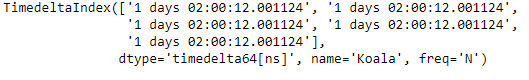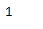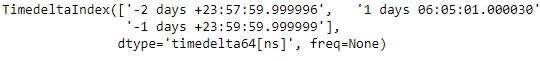Related Articles
Python | Pandas TimedeltaIndex.nlevels
• Last Updated : 29 Dec, 2018

Python is a great language for doing data analysis, primarily because of the fantastic ecosystem of data-centric python packages. Pandas is one of those packages and makes importing and analyzing data much easier.

Pandas` TimedeltaIndex.nlevels` attribute return the number of levels of the TimedeltaIndex object under consideration.

Syntax : TimedeltaIndex.nlevels

Return : number of levels

Example #1: Use `TimedeltaIndex.nlevels` attribute to find out the number of levels of the given TimedeltaIndex object under consideration

 `# importing pandas as pd``import` `pandas as pd`` ` `# Create the TimedeltaIndex object``tidx ``=` `pd.TimedeltaIndex(start ``=``'1 days 02:00:12.001124'``, periods ``=` `5``,``                                             ``freq ``=``'N'``, name ``=``'Koala'``)`` ` `# Print the TimedeltaIndex``print``(tidx)`

Output :Now we will find the number of levels of the tidx object.

 `# return the number of levels of the tidx object``tidx.nlevels`

Output :As we can see in the output, the `TimedeltaIndex.nlevels` attribute has returned 1 indicating that the number of levels of the tidx object is 1.

Example #2: Use `TimedeltaIndex.nlevels` attribute to find out the number of levels of the given TimedeltaIndex object under consideration.

 `# importing pandas as pd``import` `pandas as pd`` ` `# Create the TimedeltaIndex object``tidx ``=` `pd.TimedeltaIndex(data ``=``[``'-1 days 2 min 3us 10ns'``, ``'1 days 06:05:01.000030'``,``                                                     ``'-1 days + 23:59:59.999999'``])`` ` `# Print the TimedeltaIndex``print``(tidx)`

Output :Now we will find the number of levels of the tidx object.

 `# return the number of levels of the tidx object``tidx.nlevels`

Output :As we can see in the output, the `TimedeltaIndex.nlevels` attribute has returned 1 indicating that the number of levels of the tidx object is 1.

Attention geek! Strengthen your foundations with the Python Programming Foundation Course and learn the basics.

To begin with, your interview preparations Enhance your Data Structures concepts with the Python DS Course.

My Personal Notes arrow_drop_up